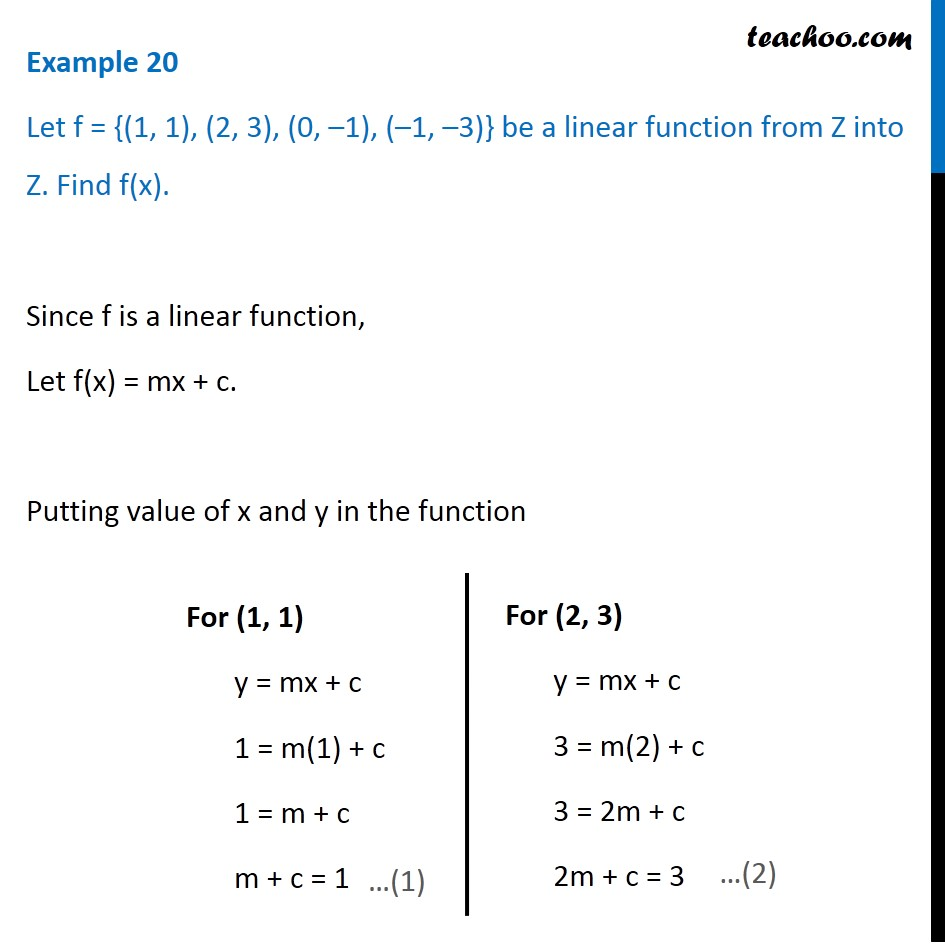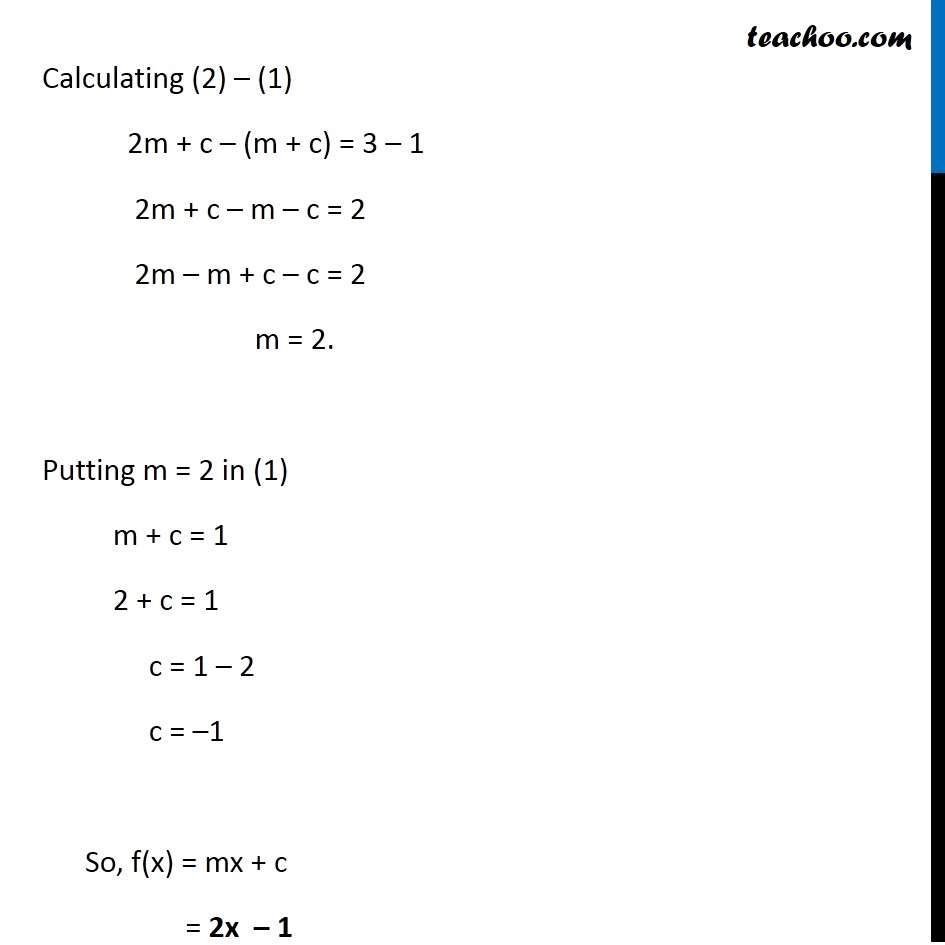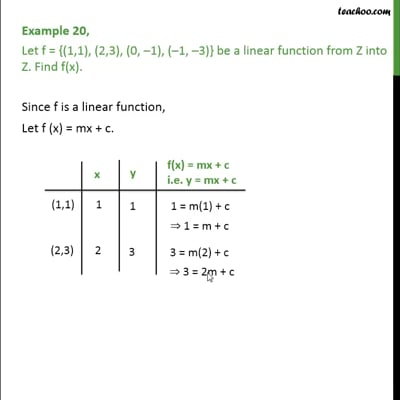Examples

Chapter 2 Class 11 Relations and Functions (Term 1)
Serial order wiseThis video is only available for Teachoo black users

### Transcript

Example 20 Let f = {(1, 1), (2, 3), (0, –1), (–1, –3)} be a linear function from Z into Z. Find f(x). Since f is a linear function, Let f(x) = mx + c. Putting value of x and y in the function For (1, 1) y = mx + c 1 = m(1) + c 1 = m + c m + c = 1 For (2, 3) y = mx + c 3 = m(2) + c 3 = 2m + c 2m + c = 3 Calculating (2) – (1) 2m + c – (m + c) = 3 – 1 2m + c – m – c = 2 2m – m + c – c = 2 m = 2. Putting m = 2 in (1) m + c = 1 2 + c = 1 c = 1 – 2 c = –1 So, f(x) = mx + c = 2x – 1